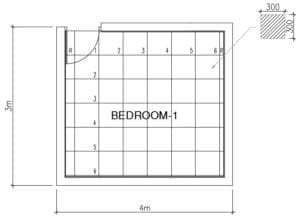# How to Calculate Tiles Needed for a Floor

To determine the number of tiles required for the given floor here are 4 simple stepsNote: Door Size = 2.1 m × 0.9 m

STEP 1

Multiply the length and breadth of that room to get the area

Total Room Floor Area = Length of the room × Breadth of the room

= 4 m × 3 m

= 12 m2

## Kitchen Designs Ideas for your Home

STEP 2

Calculate the perimeter of the room, to find skirting tiles requirement,

From the above image we can find the perimeter as

Perimeter of the room = (4 m + 3 m + 4 m + 3 m) – Door Width

= 14 m – 0.9 m

= 13.1 m

We have assumed 0.1016 m (4 inches) as skirting tiles height.

Skirting Tiles area= Perimeter of the room × Skirting Tile height

= 13.1 m × 0.1016 m

= 1.33 m2

Total Area to be laid  = Total Floor Area + Skirting Tiles area

= 12 m2  + 1.33 m2

Total Area to be laid  = 13.33 m2

## Top 13 different types of roofs with pictures

STEP 3

Calculate the area of one tile that we are planning to lay on that floor, Here We are going to use 0.3 m × 0.3 m (or 300 mm × 300 mm) tiles,

Area of one tile = 0.3 m × 0.3 m

= 0.09 (approx) m2

STEP 4

The total number of tiles required for the above room is,

Number of Tiles required = (Total Area to be laid / Area of one tile)  + Wastage*

= (13.33 m2 / 0.09 m2) + 5%

= 148.1 + 7.41 tiles

Number of Tiles required = 156 tiles (Approx)

*Wastage – Rendered wastage & damaged tiles

Therefore, we need 156 tiles, for the above room.

Assuming 1 box contain 17 tile pieces, then

Number of boxes = 156/17  = 9.2 boxes of tiles

### Check Step by Step tile  installation process

HPD Consult    info@hpdconsult.com

#### Compare

Select currency
USD United States (US) dollar
Don`t copy text!